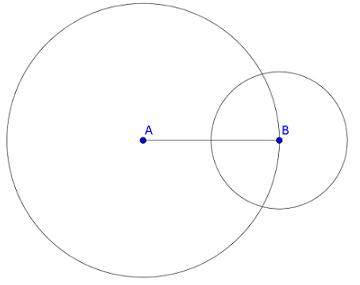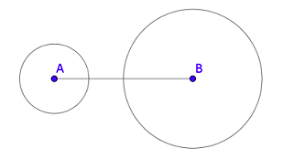Match Fishtank is now Fishtank Learning!

# Geometry

## Objective

Determine if three side lengths will create a unique triangle or no triangle.

## Common Core Standards

### Core Standards

?

• 7.G.A.2 — Draw (freehand, with ruler and protractor, and with technology) geometric shapes with given conditions. Focus on constructing triangles from three measures of angles or sides, noticing when the conditions determine a unique triangle, more than one triangle, or no triangle.

## Criteria for Success

?

1. Investigate creating triangles with different side lengths.
2. Understand why a triangle cannot be created if the sum of two side lengths is less than the third side.
3. Determine if a given set of side lengths will create a triangle.
4. Determine a measure of a third side length, given two other sides of a triangle.

## Tips for Teachers

?

The following materials are needed for this lesson: AngLegs or wooden skewers (see the blog post Triangle Inequality Skewers by Elissa Miller), compasses, rulers.

#### Remote Learning Guidance

If you need to adapt or shorten this lesson for remote learning, we suggest prioritizing Anchor Problem 1 (benefits from worked example). Students can also use the interactive geogebra tool (linked to the right) to discover the relationship As a discovery problem, if live discussion or reflection of the problem were possible, it would allow for students to arrive at the conclusion on their own. Find more guidance on adapting our math curriculum for remote learning here.

#### Fishtank Plus

• Problem Set
• Student Handout Editor
• Vocabulary Package

## Anchor Problems

?

### Problem 1

Using AngLegs or wooden skewers, investigate different side lengths that will create a triangle.

Complete the chart below, adding rows as needed.

 Side length $a$ Side length $b$ Side length $c$ Triangle? If not, explain why

What conclusion can you make about the side lengths of a triangle?

### Problem 2

In each diagram below, line segment ${AB}$  is 4 units. Two circles are drawn with a given radius and center at point $A$ and point $B$.

 Diagram 1: Circle with center $A$ has a radius of 4 units. Circle with center $B$ has a radius of 2 units.Diagram 2: Circle with center $A$ has a radius of 1 unit. Circle with center $B$ has a radius of 2 units.1. Using Diagram 1, explain or show why you can create a triangle with side lengths 4 units, 4 units, and 2 units.
2. Using Diagram 2, explain or show why you cannot create a triangle with side lengths 4 units, 1 unit, and 2 units.

## Problem Set

?The following resources include problems and activities aligned to the objective of the lesson that can be used to create your own problem set.

?

### Problem 1

Which of the following set of side lengths could not be used to create a triangle?

1. 2 cm, 6 cm, 7 cm
2. 3 cm, 3 cm, 3 cm
3. 4 cm, 5 cm, 8 cm
4. 5 cm, 7 cm, 12 cm

### Problem 2

A triangle has two side lengths that measure 6 in. and 9 in. Which of the following could be the measure of the third side? Select all that apply.

1.   3
2.   4
3.   8
4.   11
5.   14
6.   15
7.   18

?